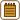## Capillary

### Task number: 2217

A capillary tube has an inner radius 0.10 mm. Evaluate:

a) When you immerse one end in water, how high will the water in the tube rise?

b) How great the hydrostatic pressure created by the water column is?

c) How does the result change when we use a capillary tube with a double radius?

d) How would the result change if we performed the experiment on the Moon?

e) How would the experiment go in a satellite in a state of weightlessness?

• #### Hint

Height of water column in the tube can be evaluated from the equilibrium of forces acting on the water in the tube. Gravity is acting down, and it is the surface force acting up that is “holding” the water in the tube.

For simplicity, let us assume that the surface force is pointing straight up and its magnitude is proportional to the inner circumference of the tube (which is the circumference of the water surface).

• #### Notation

 r = 0.1 mm = 1.0·10−4 m inner circumference of the capillary h = ? height of the water in the capillary Tabulated values: σ = 73 mN m−1 = 73·10−3 N m−1 surface tension of water ρ = 1 000 kg m−3 density of water g = 9.81 m s−2 gravitational acceleration
• #### Analysis of part a)

When solving this task we shall assume that water perfectly adheres to the tube. This means that the water surface takes on the shape of a half of a sphere and that the force given by surface tension points down. According to the third Newton’s law, glass is acting on glass with the same force of opposite direction – up. Then there is also gravitational force acting down. When water surface is in a state of equilibrium, magnitudes of both of these forces must equal. We determine the elevation difference of water from this equality.

• #### Solution of part a)

There are two forces acting upon the water: gravitational force and the force, which is glass’ reaction to surface forces.

Firstly, let’s determine the magnitude of gravitational force:

$F_G\,=\,mg\,=\,V \varrho g\,=\,\pi r^2h \varrho g\,.$

In previous equation, we used water density to express mass, and a formula for cylinder volume.

Now we determine force given by surface tension. This force acts on inner circumference o of the capillary, so for its magnitude following applies:

$F\,=\,\sigma o \,=\,\sigma 2\pi r\,.$

The magnitudes of both forces must equal:

$F_G \,=\, F$ $\pi r^2h \varrho g \,=\, \sigma 2\pi r$

Now we express h from the last equation, and input given values:

$h \,=\,\frac{ 2\sigma}{r \varrho g} \,=\,\frac{ 2\,\cdot\,0.073}{1.0\,\cdot\,10^{-4}\,\cdot\,1000\,\cdot\,9.81}\,\mathrm{m}$ $h \,=\,0.149 \,\mathrm{m}\,\dot=\,15\,\mathrm{cm}$
• #### Solution of parts b) – e)

b) Hydrostatic pressure of water column in the tube can be expressed and evaluated

$p\,=\,h\varrho g \,=\,\frac{ 2\sigma}{r \varrho g}\,\varrho g \,=\,\frac{ 2\sigma}{r }$ $p\,\dot=\,1.5\,\mathrm{kPa}$

This pressure corresponds to the so-called capillary pressure generated under the curved surface of the liquid as a result of surface tension. The previous task could be solved by equilibrium of hydrostatic and capillary pressure.

c) The height of the water in the capillary is inversely proportional to the radius of the capillary, so in a capillary with a double radius it will only reach half the height. ...

$h_b \,=\,\frac{ 2\sigma}{r_b \varrho g}\,=\,\frac{ 2\sigma}{2r \varrho g} \,=\,\frac{ 1}{2 }\,h$

d) Gravitational acceleration is six times smaller on the Moon. Height of the water in the capillary is inversely proportional to this acceleration, so the water height would be six times greater.

$h_d \,=\,\frac{ 2\sigma}{r_b \varrho g_{Mesic}}\,=\,\frac{ 2\sigma}{r \varrho \frac{ g}{6 }} \,=\,6h$

e) In a state of weightlessness, there would be no force acting upon water, so water would, due to its wettability, reach the top of arbitrarily long capillary.

• #### Answer

In the given capillary the water will rise to the height of approximately 15 cm, and water column will cause hydrostatic pressure 1.5 kPa.

In a capillary with the double radius, water would rise to the half of the height. However, on the Moon the height would be six times greater.

In a state of weightlessness, water would fill arbitrarily long capillary.